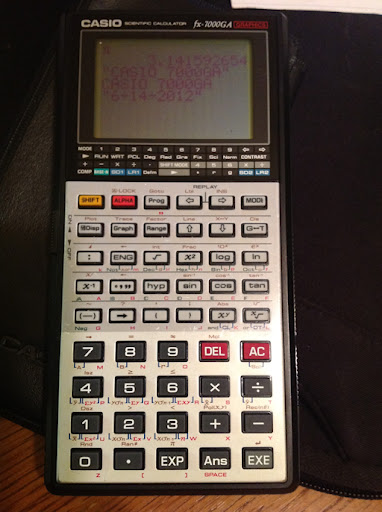## Sunday, June 17, 2012

### Casio Program Library Part 1

Here is a list of programs I did over the course of the last few days (since 6/14/2012). These programs were done using the Casio fx-6300G and fx-7000GA calculators. These programs can be ported to almost any Casio calculator that has programming.

Note: for the fx-3650P, there are no strings, graphics, and variables are limited to A, B, C, D, X, Y, and M.

If you need summary of the programming commands, check out this page.

Programs are listed in blue, Courier font. Enjoy! EddieBinomial Distribution

B
Σ N nCr K × P^K × (1-P)^(N-K) = M
K=A

A = lower limit
B = upper limit
P = probability of success (0 ≤ P ≤ 1)
M = total probability

For a single point, enter A and B as the same value

**You can shave off steps by pre-storing all the variables before execution and leaving out some the beginning prompt commands. I do that in some of these programs.

"A"?→A:"B"?→B:"N"?→N:"P"?→P:
0→M:Lbl 1:
M+(N!×P^A×(1-P)^(N-A))÷(A!(N-A)!)→M:
A+1→A:A>B⇒Goto 2:Goto 1:
Lbl 2:M

91 steps

Roots of a Complex Number

Uses the polar form of the complex number x+yi to find the n roots of it.

Note: fx-6300G and fx-7000G Rectangular and Polar Conversions

Pol(x,y) stores r in I and θ in J.
Rec(r,θ) store x in I and y in J.

For the fx-9000s series (i.e. fx-9860), lists are returned. Adjust accordingly.

(x+yi)^1/n = r^1/n cos((θ + 2k π)/n) + i r^1/n sin((θ + 2k)/n)

"X"?→X:"Y"?→Y:"N"?→N:
Pol(X,Y:Lbl 1:"RE"◢
N x√ I × cos((J+2K π ) ÷ N ◢
"IM" ◢ N x√ I sin((J+2K π ) ÷ N ◢
1 + K → K ◢ K < N ⇒ Goto 1

89 steps

Horizontal Curves

L = R θ
C = 2 R sin(θ/2)

where L=length of arc and C=chord length

Let T be θ.

"R"?→R: "T"?→T:
"C" ◢ 2R sin(T ÷ 2

34 steps

Lens Equation: Image Length and Magnitude

O^-1 +I^-1 = F^-1
M = F (F-O)^-1

Where
O = object length
F = focal length
I = image length
M = magnification

Notes:

Focal Length
F<0 for concave lens and convex mirrors (diverging light)
F>0 for convex lens and concave mirrors (converging light)

Magnification
M>0 image is upright
M<0 image is upside down
0<|M|<1 image is shrunk
|M|>1 image is enlarged

"O"?→O: "F"?→F:
"I" ◢ (F^-1 - O^-1)^-1 ◢
"M" ◢ F(F-O)^-1

38 bytes

Ellipse

With semi-axis of lengths X and Y:

Area = π X Y

Perimeter ≈ 2 π √ ((X^2 + Y^2) / 2)
(best when X and Y are close, this came from the Fundamentals of Engineering Handbook)

"X"→ X: "Y"→ Y:
"P" ◢ 2 π √ (.5 (X^2+Y^2→ P ◢
"A" ◢ π XY → A

42 steps

2 by 2 Matrices: Determinant, Inverse, Solution

[[A B][C D]] [[X][Y]] = [[E][F]]

inverse = [[D/Z -B/Z][-C/Z A/Z]] = [[G H][I J]]

solution = M^-1 b = [[G H][I J]] [[E][F]] = [[X][Y]]

If Z = 0, the matrix is singular and only the determinant is displayed.

Store A, B, C, D, E, and F before running the program

"DET" ◢
AD-BC→Z ◢ Z = 0 : Goto 1 : "INV" ◢
D ÷ Z → G ◢ -B ÷ Z → H ◢ -C ÷ Z → I ◢
A ÷ Z → J ◢ "X,Y" ◢ GE + HF → X ◢
IE + JF → Y

74 steps

Picking Random Numbers - Repeats are Allowed

N random numbers from A to B, with repeats allowed

"A"?-> A: "B"? → B:
"N"?→ N: Lbl 1: Int((B-A+1)Ran#) ◢ Dsz N : Goto 1

40 steps

There will be a Part 2 - which I will aim to post during the next week. Until then,

Eddie

This blog is property of Edward Shore. © 2012

### Casio fx-CG50: Sparse Matrix Builder

Casio fx-CG50: Sparse Matrix Builder Introduction The programs can create a sparse matrix, a matrix where most of the entries have zero valu...